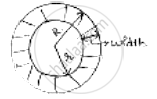Share

# The Outer Circumference of a Circular Race Track is 528m. the Track is Everywhere 14m Wide. Calculate the Cost of Leveling the Track at Rate of 50 Paise per Square Metre. - CBSE Class 10 - Mathematics

ConceptProblems Based on Areas and Perimeter Or Circumference of Circle, Sector and Segment of a Circle

#### Question

The outer circumference of a circular race track is 528m. The track is everywhere 14m
wide. Calculate the cost of leveling the track at rate of 50 paise per square metre.

#### SolutionLet inner radius = r      width(d) = 14m

Outer circumference of track = 2 𝜋𝑟

∴ 2 𝜋𝑟 = 528

2 ×22/7× R = 528 ⇒ R =528×7/2×22= 84 m

Inner radius r = R – d = 84 – 14 = 70m

Area of track = (area of outer circle) – (area of inner circles)

= 𝜋𝑅2 − 𝜋𝑟2

= pi(R^2 − r^2) =22/7(84^2 − 70^2)

=22/7(84 + 70)(84 − 70) =22/7× 154 × 14

= 6776 𝑚2

Cost of leveling 𝑚2 = 𝑅𝑠. 0.50

Total cost of leveling track = 6776 ×1/2= 𝑅𝑠. 3388

Is there an error in this question or solution?

#### Video TutorialsVIEW ALL 

Solution The Outer Circumference of a Circular Race Track is 528m. the Track is Everywhere 14m Wide. Calculate the Cost of Leveling the Track at Rate of 50 Paise per Square Metre. Concept: Problems Based on Areas and Perimeter Or Circumference of Circle, Sector and Segment of a Circle.
S